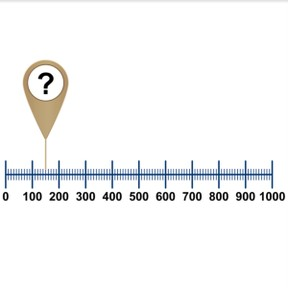Number line to 1,000 with random numbers

# Number line to 1,000 with random numbers

Number line to 1,000 with random numbers

No account needed.8,000 schools use Gynzy92,000 teachers use Gynzy1,600,000 students use Gynzy

## General

Students learn to place random numbers to 1,000 on the number line. They count forwards and backwards to place the numbers.

2.NBT.A.2

## Relevance

Discuss with students that it is important to know how to place numbers on the number line so that they know where a number belongs. They can use this to determine if a number is bigger or smaller.

## Introduction

The interactive whiteboard shows a number sequence in which students need to count backwards. They then need to draw a line from a given number to its place on the number line. Discuss that you first need to place the hundreds on the number line, before you can place numbers accurately on the number line. Erase the boxes to show the hundreds on the number line.

## Development

Explain that every mark on a number line represents a number. On a number line from 0 to 1,000 you first need to know how the numbers are represented on the number line, before you can figure out which number is indicated by a question mark. Number lines can be represented in different ways. Show the students a number line with the hundreds marked, and 10 marks between the hundreds. Say that each smaller mark represents a tens number that sits between the hundreds. Explain the other number lines. Explain that to place a number, you must first determine which hundreds a number is closest to and then count forwards or backwards from that number. Next practice with a number line that only has 0 and 1,000 marked. Tell students that they first must fill in the hundreds. Show other number lines and explain that sometimes the tens and sometimes the ones are shown with marks as well. The longer marks represent the bigger numbers. Have students practice placing numbers on different kinds of number lines. Have students determine which numbers belong with the question marks.

Check that students understand number line to 1,000 with random numbers by asking the following questions:
- How do you place a number on the number line?
- Where do you start counting?
- When do you count forwards and when do you count backwards?

## Guided practice

Students practice placing numbers on the number line using counting forwards and counting backwards. They practice on number lines with different formats. The number lines range from showing hundreds, showing tens, and showing ones. Students have multiple choice questions as well as open questions where they must determine the number themselves.

## Closing

Discuss with students that it is important to be able to place numbers on the number line so you know where a number belongs, and that recognizing differences in number lines is also important for knowing how to read a number line. There are number lines with hundreds, tens, and ones. Check that students know to count forwards or backwards from the closest hundreds, tens, or ones to a given number. To check that students understand this, show students two number lines on the interactive whiteboard and have students determine which number line shows 369.

## Teaching tips

Students who have difficulty placing random numbers on the number line to 1,000, can be supported by practicing counting forwards and backwards to 1,000 as well as practicing skip counting in 50s and 100s.

### The online teaching platform for interactive whiteboards and displays in schools

• Save time building lessons

• Manage the classroom more efficiently

• Increase student engagement